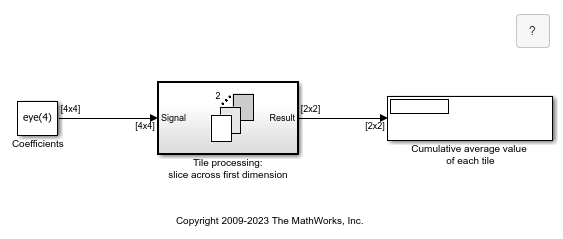# Tiled Processing of 2-D Signals with For-Each Subsystem

This example shows how to use a for-each subsystem. In this example, the operations are performed on matrices.

Open the example model named `sldemo_foreach_tiled`. This model contains a for-each subsystem that processes submatrices of a matrix input signal.To process submatrices of a matrix signal, you will need to use nested for-each subsystems. The outer subsystem can be used to partition the signal along the first dimension, while the inner one can be used to partition along the second dimension. This order is arbitrary and can be changed without affecting the result.

In this example, a four-by-four matrix is to be partitioned into two-by-two submatrices. The outer subsystem partitions the signal along the first dimension. The resulting subarrays are then partitioned by the inner subsystem along the second dimension. Concatenation of the outputs respects the partitioning order to preserve the shape of the input signal. However, the output dimensions change, since the processing of the submatrices results in scalar outputs.

To see a tile repetition implementation for this model, open the example model named `sldemo_foreach_repeat_tile`.This implementation does not use the for-each subsystem. Instead, this implementation mimics the functionality of the for-each subsystem by replicating the subsystem as many times as necessary and by selecting and concatenating the signals appropriately. This replication process is prone to error and is not scalable. Changing the input signal size would require changing the model accordingly.